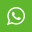## Material Content for QUESTION WITH ANSWER SET-2

यहाँ पे CCC NIELIT के महत्वपूर्ण प्रश्न जवाब के साथ दिए गए हे, अंत में सभी प्रश्न के बाद निचे इसके जवाब भी दिए गए हे ध्यान से पढ़े

41      You can change the margins in Word by

(A)using the ruler or

(B)using the page setup

(C)Both (a) and (b)

(D)None of the above

42      You can create a template in Word

(A)based on an existing document

(B)based on an existing template

(C)from scratch

(D)All the above

43      "In which Word menu, Letter wizard command appears"

(A)Format     (B)Tools        (C)Insert       (D)Table

44      The sheet tab of the Letter wizard in Word are

(A)Letter Format      (B)Recipient Info      (C)Both (a) and (b)   (D)None of the above

45      One of the following statements in not true.

(A) Word allows you to use the formula command to perform  simple calculation in a table.

(B) "The simple calculation in a table is that of addition,  subtraction, multiplication, division etc."

(C) =SUM(above) formula means that word would sum the values in  all cells above the current cell.

(D) Word cannot do simple calculation. For this purpose you   would use Excel.

46      "While using the Mail Merge Helper, you click on the Active Window button. This selection creates"

(A)a master document in the currently active document window

(B)opens a new document window

(C)your document gets active and automatically attaches to a  data source

(D)None of the above

47      Palettes that can be tear-off are

(A)LineColour (B)FillColour   (C)FontColour (D)All of the above

48      A worksheet can be opened by clicking on the

(A)Start button         (B)Open button        (C)Both a and b        (D)None of the above

49      To select a column the easiest method is to _____.

(A)double-click any cell in the column

(B)drag from the top cell in the column to the last cell in  the column

(D) [Ctrl]+{A]

50 Ms word offers certain ways by which you can move around in a document

(A)by scrolling

(B)by moving to a specific page

(C)both a and b above

(D)None of the above

51      "As long as you have your product key, you can reinstall Windows XP using any CD, even one you borrow from a friend or neighbor."

(A)True         (B)False

52      Hybrid computer is a mixture of both digital and analog computers.      (A)True         (B)False

53      Default number of decimal points can be changed with the Increase Decimal and Decrease Decimal buttons.

(A)True         (B)False

54      "To have Microsoft Excel always use a specific currency symbol, change the currency symbol selected in Regional Settings in Control Panel before you start Excel."

(A)True         (B)False

55      The name of a cell or range can be same as the cell/range reference.  (A)True         (B)False

56      =SUM(Sheet2:Sheet13!B5) adds all the values contained in cell B5 on all the worksheets between and including Sheet 2 and Sheet 13

(A)True         (B)False

57      "If one forgets to put the closing parenthesis in a formula and press [Enter] key, Excel gives an error message."

(A)True         (B)False

58      "To display the current time, the TIME() function can be used."

(A)True         (B)False

59      3-D charts have a category (x) axis a value (y) axis and a third (z) axis         (A)True         (B)False

60      Pie chart can represent multiple series of data.

(A)True      (B)False

61      Bubble chart can represent three variable on a two dimensional chart.  (A)True         (B)False

62      It is possible to undo the deletion of a sheet.

(A)True         (B)False

63      You can have a different header and footer on each sheet of a workbook.     (A)True         (B)False

64      It is possible to insert a sheet at the end of the work book.

(A)True         (B)False

65      The Slide Sorter View button automatically sorts the slides alphabetically.

(A)True         (B)False

66      "If your text exceeds the size of the placeholder, Microsoft PowerPoint reduces the font size and line spacing incrementally as you type, to make the text fit."

(A)True         (B)False

67      You can only split one cell of a table in a PowerPoint slide at a time.     (A)True         (B)False

68      Press F5 key to go to Slide Show view

(A)True         (B)False

69      Different elements in a chart cannot have different transition.

(A)True         (B)False

70      Lycos is an Internet search engine and web portal.

(A)True         (V)False

71      The act of exploring Web is known as surfing

(A)True         (B)False

72      The e-mail component of Internet Explorer is called MSN Messenger

(A)True         (B)False

73      Netscape Messenger is the e-mail client packaged with Netscape Communicator 4.0

(A)True         (B)False

74      Usenet and Internet are the same thing

(A)True         (B)False

75      The data to be drawn as a chart cannot be selected after the chart wizard is started.

(A)True         (B)False

76      Word Templates have .dot extension.

(A)True         (B)False

77      Microcomputer is called micro because it consists of micro-processor.  (A)True         (B)False

78      BIOS stands for Basic Integrated Operating System

(A)True         (B)False

79      A smart terminal has in-built processing capability.

(A)True         (B)False

80      Fetching of an instruction for execution is done by the control unit.      (A)True         (B)False

41=  C    42=  D    43=  B    44=  C    45=  D    46=  A    47=  D

48=  B    49=  C    50=  C   51=  TRUE 52=  TRUE    53=  TRUE

54=  TRUE    55=  TRUE    56=  TRUE    57=  FALSE   58=  TRUE
59=  TRUE 60=  TRUE

61=    TRUE    62=    FALSE    63=    TRUE    64=    TRUE

65=    FALSE    66=    TRUE    67=    TRUE    68=    TRUE

69=    FALSE    70=   TRUE    71=    TRUE    72=    TRUE

73=    TRUE    74=    FALSE    75=    FALSE    76=    FALSE

77=    TRUE    78=    FALSE    79=    TRUE   80=    TRUE

## View MoreMaterial

### Share

#### .share-buttons{ list-style: none; } .share-buttons li{ display: inline; }